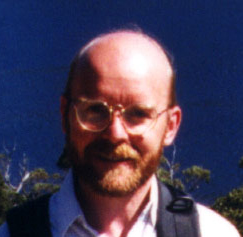# DR CRAIG HODGSON

## TOPOLOGY AND GEOMETRY

 Home/Research Interests Teaching Publications Undergraduate Projects Computational Tools

I am especially interested in geometry and topology . My main research is in low-dimensional topology, which is a very active and fertile area of current mathematical research with many long standing open questions. I am particularly interested in the application of geometric methods to study the topology of 3-dimensional manifolds.

A 3-dimensional manifold, or "3-manifold", is a space that looks locally like Euclidean 3-dimensional space in the same way that the surface of a sphere or doughnut, for example, looks locally (to a small inhabitant) like a flat plane. Three-dimensional manifolds are of fundamental significance in theoretical mathematics, especially in topology and algebra. They are also of importance in cosmology, providing models for our universe. In fact, astronomers and mathematicians are currently analysing new satellite data on the variation in cosmic microwave background radiation to try to determine the size and global topology of our universe.

Thurston's "Geometrization Conjecture" gives a beautiful conjectural classification of 3-manifolds in terms of eight uniform geometries, but the conjecture and some of its basic consequences remained unproved for over 25 years. My work has advanced our understanding by proving the Geometrization Conjecture in several important new cases. Recently the Geometrization Conjecture was proved by Grigori Perelman using the Ricci curvature flow developed by Richard Hamilton.

The most important kind of geometry for understanding 3-manifolds is hyperbolic geometry: this is the non-Euclidean geometry of constant negative curvature, where angles in triangles add up to less than 180 degrees. Thurston's work shows that ``most'' 3-manifolds are hyperbolic, and that analysing these hyperbolic 3-manifolds is crucial to understanding 3-dimensional topology. Another large part of my research has been devoted to understanding the geometric and topological properties of these hyperbolic 3-manifolds. An important aspect of this work has been the development of effective computational methods for the study of 3-manifolds.

There is a very active and enthusiastic group of staff and students in the topology and geometry area:

Past and Present Students